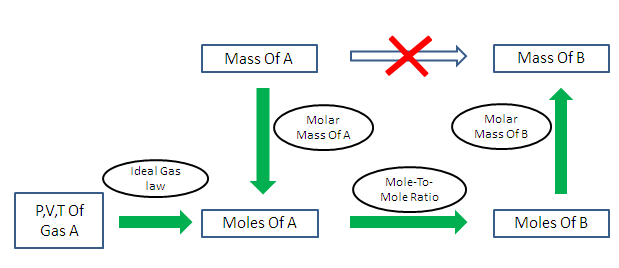# Amount of substance (n) Calculation

Calculates the amount of substance for given values.

 Mass(kg) : Molar Mass(g/mol) :

 Amount of Substance (n) : (mole)

The calculator finds the amount of substance having the number of molecules, ions, atoms or other specified group of entities present in any compound, which is the ratio of Mass / Molar mass.

The mole is fundamental unit in the SI system and it is used to measure the amount of substance.

## Formula Used:

Amount of substance (n)=Mass (m)/Molar mass (M)

Mass is the weight in kilograms, whereas molarmass is the mass of one mole of a compound in g/mol(gram/mole).